# Reference formulas and other worksheets in Excel

You don't have to have all your data on one worksheet. In fact, it's common practise to create lots of worksheets in the same workbook. In this lesson, you'll see how to reference a formula that is on a different worksheet. This comes in handy if, for example, you have 12 worksheets, one for each month of the year. You can then create another worksheet that holds things like totals for the entire year. We'll do that now.

• Open up Excel (If it's already open, close the project you're currently working on and open a new blank workbook.)
• Locate Sheet1, Sheet2, and Sheet3 at the bottom of Excel (Excel 2013 and 2016 users will only have one worksheet. To create two more worksheets, click the plus symbol to the right of Sheet1.)
• Rename these to May, June, July (Right click, and select Rename from the menu)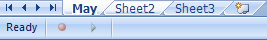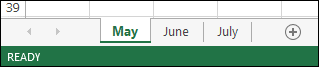Click on the May sheet, and enter the same data as in the following image:Click on the June sheet and enter the following:Then click on the July sheet and enter the following: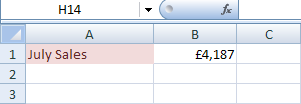We now need to create a new worksheet. So click on the New Worksheet icon at the bottom of Excel, the one to the right of July in the image below (Excel 2013 and 2016 users should click the plus symbol again):The new worksheet will be called Sheet4 by default. Rename it to Annual Total, and your workbook will look like this at the bottom (If your new worksheet is not at the end, hold down your left mouse button on the worksheets name. Keep it held down and drag to the end):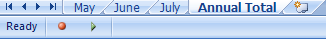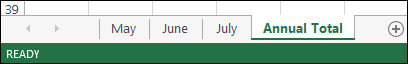We're now going to add up the figures on the May, June and July worksheets, and put the answer on the Annual Total worksheet.

Add a label to your Annual Total worksheet:Then click inside cell B1.

To reference data on another worksheet, you use the exclamation mark (exclamation point, if you're in the USA). This is commonly called a Bang!

So enter this in cell B1 of your Annual Total worksheet:

=May!B1

So we start with an equals sign (=), and then type the Name of the worksheet we want to reference (May). After the exclamation mark (bang), we have the cell we want to reference (B1). If you just type B1 by itself, Excel would assume that you meant the current worksheet.

When you press the enter key, you should see this on your Annual Total worksheet:This is the same figure as the one on your May worksheet. To add up all our monthly worksheets, just reference them in the same manner:

=May!B1 + June!B1 + July!B1

So click inside cell B1 of your Annual Total worksheet and replace your formula with the one above. Press the enter key and you should see the answer: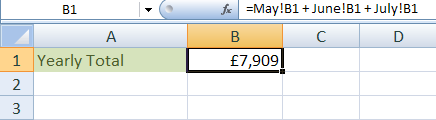So when you want to include figures or formula from other worksheets, remember to include the name of the worksheet followed by a bang

In the next part, you'll learn about the LOOKUP function in Excel.

<--Back to the Excel Contents Page